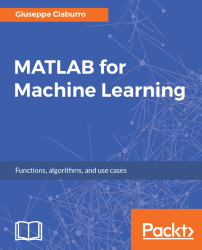•#### MATLAB for Machine Learning#### Overview of this book

MATLAB is the language of choice for many researchers and mathematics experts for machine learning. This book will help you build a foundation in machine learning using MATLAB for beginners. You’ll start by getting your system ready with t he MATLAB environment for machine learning and you’ll see how to easily interact with the Matlab workspace. We’ll then move on to data cleansing, mining and analyzing various data types in machine learning and you’ll see how to display data values on a plot. Next, you’ll get to know about the different types of regression techniques and how to apply them to your data using the MATLAB functions. You’ll understand the basic concepts of neural networks and perform data fitting, pattern recognition, and clustering analysis. Finally, you’ll explore feature selection and extraction techniques for dimensionality reduction for performance improvement. At the end of the book, you will learn to put it all together into real-world cases covering major machine learning algorithms and be comfortable in performing machine learning with MATLAB.
Title PageCreditsForewordwww.PacktPub.comCustomer FeedbackPrefaceFree Chapter
Getting Started with MATLAB Machine LearningImporting and Organizing Data in MATLABFrom Data to Knowledge DiscoveryFinding Relationships between Variables - Regression TechniquesPattern Recognition through Classification AlgorithmsIdentifying Groups of Data Using Clustering MethodsSimulation of Human Thinking - Artificial Neural NetworksImproving the Performance of the Machine Learning Model - Dimensionality ReductionMachine Learning in Practice## Probabilistic classification algorithms - Naive Bayes

Bayesian classification is a statistical technique that determines the probability that an element belongs to a particular class. For example, this technique can be used to estimate the probability of a customer belonging to the class of sports car buyers, given some customer attributes such as type of work performed, age, income, civil status, sports practiced, and so on.

The technique is based on the theorem of Bayes, a mathematician and British Presbyterian minister of the eighteenth century. The theorem defines the posterior probability of an event with respect to another. Posterior, in this context, means after taking into account the events relevant to the particular case being examined as if they have already happened.

The Bayesian classifier algorithm assumes that the effect of an event on a given class is independent of the values ​​of other events. This assumption, called the conditional independence of the classes, is intended...